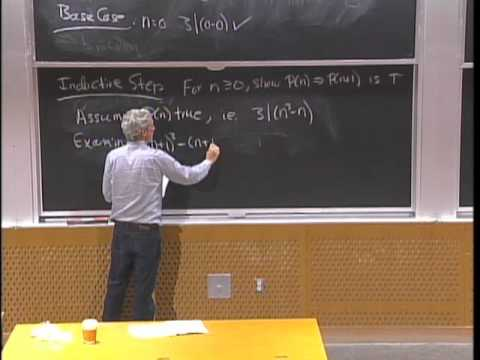MIT OpenCourseWare

# Mathematics for Computer Science

This course covers elementary discrete mathematics for computer science and engineering. It emphasizes mathematical definitions and proofs as well as applicable methods. Topics include formal logic notation, proof methods; induction, well-ordering; sets, relations; elementary graph theory; integer congruences; asymptotic notation and growth of functions; permutations and combinations, counting principles; discrete probability. Further selected topics may also be covered, such as recursive definition and structural induction; state machines and invariants; recurrences; generating functions. (from ocw.mit.edu )

Instructor

Computer science
2012

#### Lecture 02 - Induction